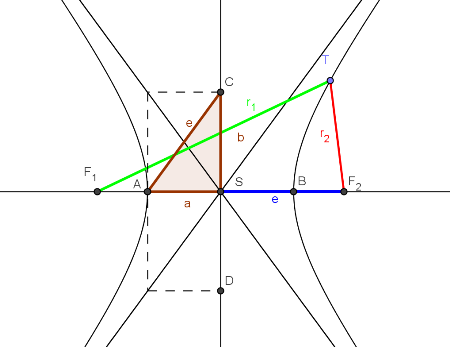## Hyperbola

Hyperbola is the locus of points where the absolute value of the difference of the distances to the two fixed points is a constant equal to 2a, the distance between its two vertices.F1, F2 - fixed points: foci or focal points of the hyperbola A, B - vertices of the hyperbolaS - center of the hyperbola 2a=|AB| - transverse or major axis 2b=|CD| - conjugate or minor axise - linear eccentricitye2 = a2 + b2 r1, r2 - radial vectors of the point T

Created by Helena Halas, translated by Helena Halas and Iva Kodrnja - 3DGeomTeh - Developing project of the University of Zagreb